# Previous Year Questions: Chemical Reactions & Equations Notes | Study Science Class 10 - Class 10

## Class 10: Previous Year Questions: Chemical Reactions & Equations Notes | Study Science Class 10 - Class 10

The document Previous Year Questions: Chemical Reactions & Equations Notes | Study Science Class 10 - Class 10 is a part of the Class 10 Course Science Class 10.
All you need of Class 10 at this link: Class 10

Q 1. 2 g of silver chloride is taken in a china dish and the china dish is placed in sunlight for sometime. What will be your observation in this case? Write the chemical reaction involved in the form of a balanced chemical equation. Identify the type of chemical reaction.    [Delhi 2019]

The colour of salt will change from white to grey.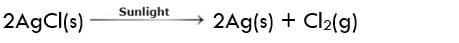It is a photochemical decomposition reaction.

Q 2. Decomposition reactions require energy either in the form of heat or light or electricity for breaking down the reactants. Write one equation each for decomposition reactions where energy is supplied in the form of heat, light and electricity.    [CBSE 2018]

(i) Energy supplied in the form of heat
Decomposition of ferrous sulphate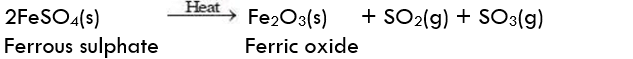(ii) Energy supplied in the form of light:
Decomposition of silver chloride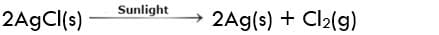(iii) Energy supplied in the form of electricity:
Electrolysis of water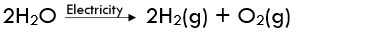Q 3. In the reaction:   [CBSE 2018C]
MnO2 + 4HCl → MnCl2 + 2H2O + Cl2
(a) Name the compound

(i) Oxidised
(ii) Reduced

(b) Define oxidation and reduction on its basis

(a) (i) HCL is getting, oxidised.
(ii) MnO2 is getting reduced.
(b) The removal of hydrogen from a substance is called oxidation. The removal of oxygen from a substance is called reduction.

Q 4. Classify the following chemical reactions as exothermic or endothermic:    [CBSE 2017]
(i) Water is added to quick lime.
(ii) Dilute sulphuric acid is added to zinc granules.
(iii) When ammonium chloride is dissolved in water in a test tube it becomes cold.
(iv) The decomposition of vegetable matter into compost.
(v) Electrolysis of water.
(vi) Silver chloride turns grey in the presence of sunlight to form silver metal.

(i) Water is added to quick lime - Exothermic
(ii) Dilute sulphuric acid is added to zinc granules - Exothermic
(iii) When ammonium chloride is dissolved in water in a test tube it becomes cold - Endothermic
(iv) The decomposition of vegetable matter into compost - Endothermic
(v) Electrolysis of water - Endothermic
(vi) Silver chloride turns grey in the presence of sunlight to form silver metal - Endothermic

Q 5. Name the type of chemical reaction represented by the following equations:    [CBSE 2017]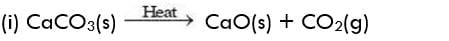(ii) CaO(s) + H2O(l) → Ca(OH)(aq)
(iii) Zn (s) + H2SO4 → ZnSO4(aq) + H2(g)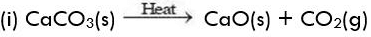This is a decomposition reaction.
(ii) CaO (s) + H2O(l) → Ca(OH)2 (aq)
This is a combination reaction.
(iii) Zn(s) + H2SO4 → ZnSO4 (aq) + H2(g)
This is a displacement reaction.

Q 6. You must have tasted or smelt the fat-containing food material left for a long time. Such foods taste and smell bad. What is the reason for this? Name the phenomenon responsible for it. List two measures for its prevention.    [CBSE 2016]

Food material left for a long time is oxidized by atmospheric oxygen. This changes the smell and taste of food.
Measures for prevention:
(i) Keep the food in air-tight containers.
(ii) Flush the food packets with nitrogen gas before sealing.

Q 7. Write chemical equations for the reactions taking place when:    [CBSE 2016]
(i) Magnesium reacts with dilute HNO3.
(ii) Sodium reacts with water.
(iii) Zinc reacts with dilute hydrochloric acid.

(i) Mg + 2HNO3 (dil.) → Mg(NO3)2 + H2
(ii) 2Na + 2H2O → 2NaOH + H2
(iii) Zn + 2HCl → ZnCl2 + H2

Q 8. Take 3 g of barium hydroxide in a test tube, now add about 2g of ammonium chloride and mix the contents with the help of a glass rod. Now touch the test tube from outside.    [CBSE 2016]
(i) What do you feel about touching the test tube?
(ii) State the inference about the type of reaction that occurred.
(iii) Write the balanced chemical equation of the reaction involved.

(i) The test tube becomes hot.
(ii) It is a decomposition reaction.
(iii) 2NH4Cl + Ba(OH)2 → BaCl2 + 2NH3↑ + 2H2O

Q 9. Balance the following chemical equations : [CBSE 2016]
(a) Mg + N2 → Mg3N2
(b) Al + Cl2 → AlCl3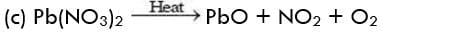(a) 3Mg + N2 → Mg3N2
(b) 2Al + 3Cl2 → 2AlCl3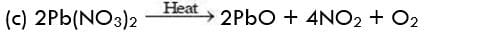Q 10. Define the term decomposition reaction. Give one example each of thermal decomposition and electrolytic decomposition.    [CBSE 2016]

Those reactions in which a compound splits up into two or more simpler substances are called decomposition reactions.
Thermal decomposition: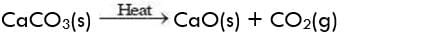Electrolytic decomposition: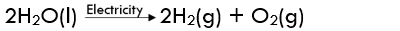Q 11. Look at the figure given below and answer the following questions:    [CBSE 2015]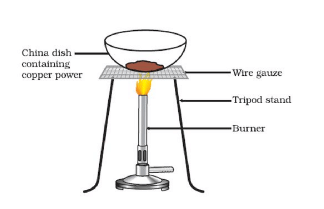(i) State the colour of the reactant and the product of the chemical reaction.
(ii) Write the chemical equation involved in this process.
(iii) Can we convert the product obtained back to reactant? Write the reaction involved.

(i) Colour of reactant: Reddish brown
Colour of product: Black(iii) On passing H2 gas over hot CuO, the reaction can be reversed.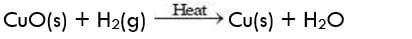Q 12. (a) Write the essential condition for the following reaction to take place:    [CBSE 2015]
2AgBr → 2Ag + Br2
Write one application of this reaction.
(b) Complete the following chemical equation of a chemical reaction: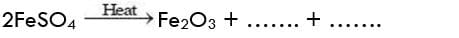(c) What happens when water is added to quick lime? Write a chemical equation.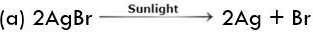• The presence of sunlight is necessary for the reaction to take place.
• This reaction is used in black and white photography.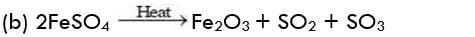(c) A hissing sound is heard. A brisk reaction takes place and calcium hydroxide is formed. A lot of heat is also produced
CaO + H2O → Ca(OH)2

Q 13. State reason for the following:    [CBSE 2015]
(i) Potato chip manufacturers usually flush bags of chips with nitrogen gas.
(ii) Iron articles lose their shine gradually.
(iii) Foods should be kept in air-tight containers.

(i) Potato chips contain oils and fats, which react with atmospheric oxygen and become rancid. To protect chips from oxidation, nitrogen gas is passed through bags of chips which removes air (oxygen) from them. Nitrogen does not react with chips.
(ii) Iron article on keeping in the open for a long time reacts with oxygen and moisture and forms the rust on the surface. Articles lose their shine because of this.
(iii) Foods kept in the open are oxidised by the oxygen in the air. Their taste and smell change. When kept in air-tight containers, the food does not come into contact with air and is not spoilt.

Q 14. State the type of chemical reactions with chemical equations that take place in the following:    [CBSE 2015]
(a) Magnesium ribbon is burnt in the air.
(b) Electric current is passed through water.
(c) Ammonia and hydrogen chloride gases are mixed.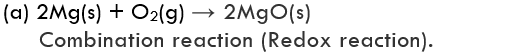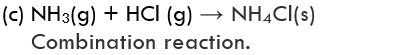Q 15. Name the type of chemical reaction represents by the following equation:    [CBSE 2015]
(a) CaO + H2O → Ca(OH)2
(b) 3BaCl2 + Al2(SO4)3 → 3BaSO4 + 2AlCl3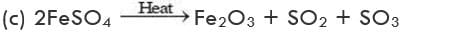(a) Combination reaction.
(b) Double displacement reaction (Precipitation)
(c) Decomposition reaction.

Q 16. (a) Consider the following chemical reaction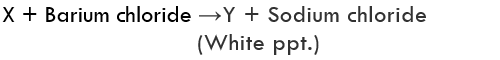(i) Identify ‘X ’ and ‘Y ’.
(ii) The type of reaction.    [BSE 2015]
(b) Identify the type of reaction from the following equations:

(i) HCl(aq) + NaOH(aq) → NaCl(aq) + H2O(l)
(ii) Fe(s) + CuSO4(aq) → FeSo4(aq) + Cu(s)

(a)
(i) ‘X’ Is Na2SO4 and Y is BaSO4
(ii) Na2SO4 + BaCl2 → BaSO4 + 2NaCl
(White ppt.)
The reaction is a precipitation reaction. It is also called a double displacement reaction.
(b)
(i) Double displacement reaction.
(ii) Displacement reaction.

Q 17. 2g of ferrous sulphate crystals are heated in a dry boiling tube.

[CBSE 2015, 2012]
(a) List any two observations.
(b) Name the type of chemical reaction taking place.
(c) Write the chemical equation for the reaction.

(a) Two observations are as follows:
(i) Green colour of FeSO4 disappears and reddish-brown Fe2O3 is formed.
(ii) Smell of burning sulphur.
(b) Decomposition reaction.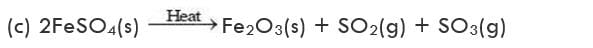Q 18. Write the chemical equation of the reaction in which the following changes have taken place with an example of each:    [CBSE 2015,2014,2011]
(a) change in colour.
(b) change in temperature.
(c) formation of a precipitate.

(a) Cu(s) + 2AgNO3(aq) → Cu(NO3)2(aq) + 2Ag
The solution will become blue in colour and shiny silver metal will be deposited.
(b) NaOH + HCl → NaCl + H2O + Heat
The temperature will increase because heat will be evolved.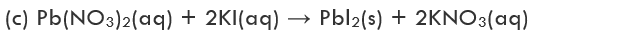Yellow precipitate of PbI2 will be formed.

Q 19. Balance the following chemical equation:    [CBSE 2015]
NaOH + H2SO→ Na2SO4 + H2O

The balanced chemical equation is:
2NaOH + H2SO4 → Na2SO4 + 2H2O

Q 1. (a) What is a balanced chemical equation? Why should chemical equations be balanced?
(b) Write the chemical equation of the reaction in which the following changes have taken place:    [CBSE 2017 C]
(i) Change in colour
(ii) Change in temperature
(iii) Formation of a precipitate.

(a)

• A chemical equation in which the total mass of the reactants is equal to the total mass of the products is called a balanced chemical equation.
• It may also be defined as a chemical equation in which the number of atoms of each element is equal on the reactant side as well as on the product side.
• It is only after balancing the chemical equation that we can find out how much of each product will be formed from certain quantities of the reactants, in terms of law of conservation of mass.

(b) Chemical equations corresponding to different changes are given below:
(i) Change in colour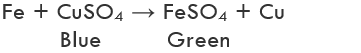(ii) Change in temperature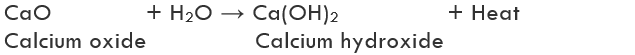(iii) Formation of a precipitate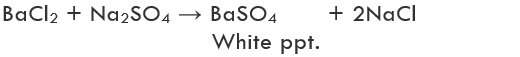Q 2. (a) Balance the following chemical equations:    [CBSE 2016]
(i) NaOH + H2SO4 → Na2SO4 + H2O
(ii) PbO + C → Pb + CO2
(iii) Fe2O3 + Al → Al2O3 + Fe + Heat
(b) Write the balanced chemical equations for the following reactions:
(i) Barium chloride + Potassium sulphate → Barium sulphate + Potassium chloride
(ii) Zinc + Silver nitrate → Zinc nitrate + Silver

(a) (i) 2NaOH + H2SO4 → Na2SO4 + 2H2O
(ii) 2PbO + C → 2Pb + CO2
(iii) Fe2O+ 2Al → Al2O3 + 2Fe + Heat
(b) (i) BaCl2 + K2SO4 → BaSO4 + 2KCl
(ii) Zn + 2AgNO3 → Zn(NO3)2 + 2Ag

Q 3. (a) Can we stir silver nitrate solution with a copper spoon? Why or why not?
(b) Why a brown coating is formed on the iron rod when the iron rod is kept dipped in copper sulphate solution for some time? What change will be observed in the colour of the solution?
(c) A green coating develops on the copper vessel in the rainy season. Why?    [CBSE 2015]

(a) We cannot stir silver nitrate with a copper spoon. Copper being more reactive than silver displaces silver from silver nitrate, that is, a reaction will take place.
(b)

• Iron being more reactive than copper displaces copper from copper sulphate by the following reaction: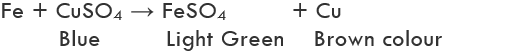• Brown coating of copper is deposited on the iron rod. Colour of the solution becomes green due to the formation of ferrous sulphate.

(c) Copper reacts with moist carbon dioxide in the air in the rainy season and a green coating is formed. This is due to the formation of copper carbonate.

Q 4. (a) Illustrate an activity along with a labelled diagram, to show that a change in the state of matter and change in temperature takes place during a chemical reaction.
(b) Write balanced chemical equations for the following reactions:
(i) Natural gas burns and combines with oxygen to produce carbon dioxide and water.
(ii) Ferrous sulphate crystals on heating break up into ferric oxide, sulphur dioxide and sulphur trioxide.    [CBSE 2015]

(a)

• Take about 5g of quick lime in a beaker and add to it about 50 mL of water. A brisk reaction takes place and a lot of heat is evolved.
CaO + H2O → Ca(OH)2 + Heat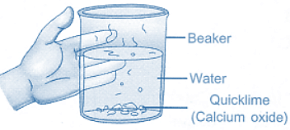Reaction of Quick Lime with Water
• Quick lime is a white solid but after the reaction, calcium hydroxide is formed which is soluble in water and a clear solution is obtained.
• Thus, there has been a change in the state of matter and a change in temperature.

(b) (i) CH4 + 2O2 → CO2 + 2H2O
(ii) 2FeSO4.7H2O → Fe2O3 + SO2 + SO+ 14H2O

Q 5. (a) Explain two ways by which food industries prevent rancidity.
(b) Discuss the importance of decomposition reaction in the metal industry with three points.    [CBSE 2015]

(a) (i) Rancidity can be prevented by adding antioxidants to food containing fat and oil. Example: Butylated hydroxy anisole is added to butter as an antioxidant.
(ii) It can be prevented by packaging fat and oil-containing foods in nitrogen gas.
(b) (i) Molten NaCl is electrolytically decomposed to form sodium metal.
(ii) Aluminium metal is obtained by the electric decomposition of bauxite ore mixed with cryolite.
(iii) Carbonate ores are thermally decomposed to give metal oxide which on reduction gives metal.

The document Previous Year Questions: Chemical Reactions & Equations Notes | Study Science Class 10 - Class 10 is a part of the Class 10 Course Science Class 10.
All you need of Class 10 at this link: Class 10Use Code STAYHOME200 and get INR 200 additional OFF

## Science Class 10

107 videos|325 docs|162 tests

Track your progress, build streaks, highlight & save important lessons and more!

,

,

,

,

,

,

,

,

,

,

,

,

,

,

,

,

,

,

,

,

,

;